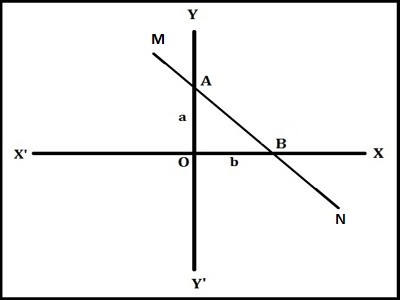Intercepts through by Straight Line on Axes

The intercepts of a line are the points where the line intercepts, or crosses, the horizontal and vertical axes. A straight line moves so that the sum of the reciprocals of its intercepts made on axes is constant.

Let a straight line ‘MN’ does not passes through the origin which meets x-axis in the point M and y-axis in the point N, then the intercept made by the line MN on x-axis is OM i.e., OM is called x-intercept.

Again, the intercept made by the line MN on y-axis is ON i.e., ON is called y-intercept

Both OM and ON called the intercepts made by the line MN on both x-axis and y-axis.

The line intercept between the axes is the line MN.Let AB be a line cutting the x-axis and the y-axis at A and B respectively on the positive sides of the axes. Let OA = a and OB = b. Then, the intercepts made on the axes of reference by the line are ‘a’ and ‘b’ respectively. So, x-intercept = a, and y-intercept = b.

Convention for the signs of intercepts:

• The x-intercept is considered positive if it is measured to the right of the origin
• The y-intercept is considered positive if it is measured above the origin
• The x-intercept is considered negative if measured to the left of the origin.
• If A is on the negative side of the x-axis, the intercept on the x-axis (i.e., x-intercept) is = -a.
• The y-intercept is considered negative if measured below the origin.
• If B is on the negative side of the y-axis, the intercept on the y-axis (i.e., y-intercept) is = -b.

Remember:

(i) When a straight line passes through origin then it makes zero intercepts on axis.

(ii) Any horizontal straight line has no x-intercept

(iii)  Any a vertical line has no y-intercept

(iii) The intercepts on x-axis is denoted by a

(iv) The intercepts on y-axis is denoted by b.

(v) When a straight line has only y-intercept, then the intercept denoted by c.

Information Source: